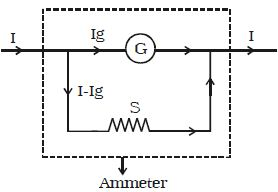Physics

# How to Conversion of Galvanometer into an Ammeter?

Conversion of Galvanometer into an Ammeter: A galvanometer is a device used to detect the flow of current in an electrical circuit. Even though the deflection is directly proportional to the current, the galvanometer scale is not marked in ampere. Being a very sensitive instrument, a large current cannot be passed through the galvanometer, as it may damage the coil. However, a galvanometer is converted into an ammeter by connecting a low resistance in parallel with it. As a result, when large current flows in a circuit, only a small fraction of the current passes through the galvanometer and the remaining larger portion of the current passes through the low resistance. The low resistance connected in parallel with the galvanometer is called shunt resistance. The scale is marked in ampere.Fig: Conversion of galvanometer into an ammeter

The value of shunt resistance depends on the fraction of the total current required to be passed through the galvanometer. Let Ig be the maximum current that can be passed through the galvanometer. The current Ig will give full scale deflection in the galvanometer.

Galvanometer resistance = G

Shunt resistance = S

Current in the circuit = I

Current through the shunt resistance = Is = (I–Ig)

Since the galvanometer and shunt resistance are parallel, potential is common.

∴ Ig x G = (I- Ig)S

S = G Ig/ (I- Ig)

The shunt resistance is very small because Ig is only a fraction of I.

The effective resistance of the ammeter Ra is (G in parallel with S)

1/Ra = 1/G + 1/S

so, Ra = GS/(G+S)

Ra is very low and this explains why an ammeter should be connected in series. When connected in series, the ammeter does not appreciably change the resistance and current in the circuit. Hence an ideal ammeter is one which has zero resistance.